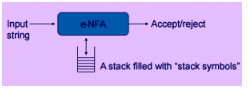Courses

# Test: From Grammars to Push Down Automata

## 10 Questions MCQ Test Theory of Computation | Test: From Grammars to Push Down Automata

Description
This mock test of Test: From Grammars to Push Down Automata for Computer Science Engineering (CSE) helps you for every Computer Science Engineering (CSE) entrance exam. This contains 10 Multiple Choice Questions for Computer Science Engineering (CSE) Test: From Grammars to Push Down Automata (mcq) to study with solutions a complete question bank. The solved questions answers in this Test: From Grammars to Push Down Automata quiz give you a good mix of easy questions and tough questions. Computer Science Engineering (CSE) students definitely take this Test: From Grammars to Push Down Automata exercise for a better result in the exam. You can find other Test: From Grammars to Push Down Automata extra questions, long questions & short questions for Computer Science Engineering (CSE) on EduRev as well by searching above.
QUESTION: 1

### The production of the form A->B , where A and B are non terminals is called

Solution:

A->ε is termed as Null production while A->B is termed as Unit production.

QUESTION: 2

### Halting states are of two types. They are:

Solution:

Halting states are the new tuple members introduced in turing machine and is of two types: Accept Halting State and Reject Halting State.

QUESTION: 3

### A push down automata can be represented as: PDA= ε-NFA +[stack] State true or false:

Solution:QUESTION: 4

A pushdown automata can be defined as: (Q, ∑, G, q0, z0, A, d)
What does the symbol z0 represents?

Solution:

z0 is the initial stack symbol, is an element of G. Other symbols like d represents the transition function of the machine.

QUESTION: 5

Which of the following correctly recognize the symbol ‘|-‘ in context to PDA?

Solution:

Using this notation, we can define moves and further acceptance of a string by the machine.

QUESTION: 6

Which among the following is true for the given statement?Statement :If there are strings R and T in a language L so that R is prefix of T and R is not equivalent to T.

Solution:

If M is a DPDA accepting L by an empty stsck, R and T are distinct strings in L, and R is a prefix of T, then the sequence of moves M must make in order to accept R leaves the stack empty, since R∈L. But then T cannot be accepted, since M cant move with an empty stack.

QUESTION: 7

Which of the following can be accepted by a DPDA?

Solution:

Theorem: The language pal of palindromes over the alphabet {0,1} cannot be accepted by any finite automaton , and it is therefore not regular.

QUESTION: 8

For a counter automaton, with the symbols A and Z0, the string on the stack is always in the form of __________

Solution:

:The possible change in the stack contents is a change in the number of A’s on the stack.

QUESTION: 9

Statement: Counter Automaton can exist for the language L={0i1i|i>=0}

Solution:

The PDA works as follows. Instead of saving excess 0’s or 1’s on the stack, we save *’s and use two different states to indicate which symbol there is currently a surplus of. The state q0 is the initial state and the only accepting state.

QUESTION: 10

Let ∑={0,1}* and the grammar G be:
S->ε
S->SS
S->0S1|1S0
State which of the following is true for the given

Solution:

A string is said to be balanced if it consist of equal number of 0’s and 1’s.